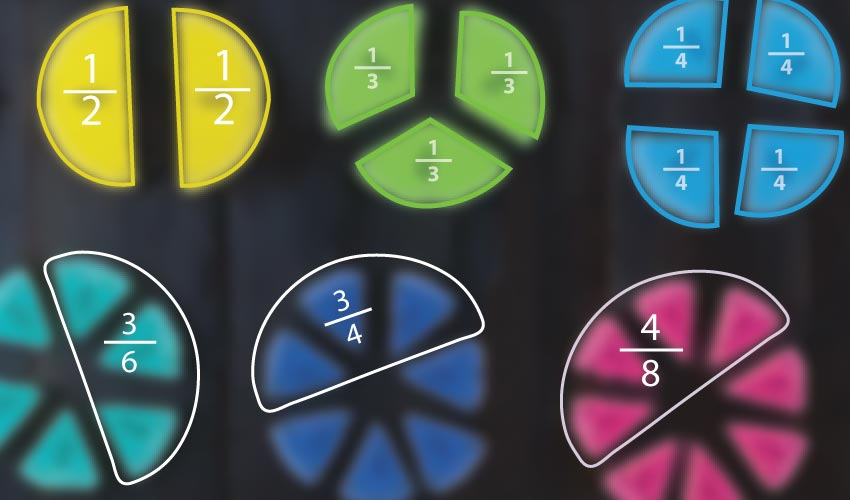# Basics of Fractions with TypesFractions are the mathematical expression of something that is divided into two or more pieces or parts. The two parts of the fractions are the denominator and the numerator. In the written expression, they are divided by a slash.

There is a lot of complicatedness presumed about fractions but they’re really not a hard nut to crack at all. Sometimes when our basics of a mathematical concept are not clear, we end up biting our nails at them throughout our lives. But this shouldn’t be the case with fractions because, in this article, we’ll discuss them as well as the basics of fractions in the simplest way to make them completely understandable for everyone.

## Fraction- What does it mean?

Let’s divide a thing into parts. Let’s just divide an apple into 4 parts. Once we are done dividing the apple, we want to give away 3 parts to someone so we are left with only one.

If we want to make a fraction out of the division that we just did then it will be 3/4th apple donated by us.

## How is a fraction written?

We just wrote a fraction in two numbers that were separated by a slash. The number before the slash is called a numerator whereas the one after the slash is referred to as a denominator. The slanted slash can be replaced by a horizontal line which divides a number on top and the other one on the bottom.

## What does a fraction tell?

A fraction simply tells us the following:

The denominator tells us exactly into how many parts is the apple divided and the numerator shows the number of slices that we have given away. Had we divided the apple into 6 parts the denominator would have been 6 instead of 4. And the half of an apple is going to be ½.

## Types of Fractions:

Now that we have understood what fractions are, let’s discuss their types. They can be classified into the following types:

1. Proper and improper fractions
2. Like and unlike fractions
3. Mixed fractions

### Proper and Improper Fractions

We know that fraction consists of two number values, the numerator one and the denominator one. If the numerator value is less than the denominator then it is referred to as a proper fraction, whereas the improper fraction is the one in which the denominator is smaller than the numerator.

For example, 3/4 is a proper fraction and 8/5 is an improper one.

An improper fraction requires the remaining parts from another piece of the same object. For example, 6/4th of an apple would mean that the remaining two apple slices were borrowed from another apple.

### Like and Unlike Fractions

Now, these are a bit tricky. Like fractions, as the name suggests, are those, that have the same value. For example, the fractions 4/6 and 8/12 are like fractions because both the fractions are 2/3rd of a thing in their simplified form. They are also called equivalent fractions.

Contrary to the above, unlike fractions are different fractions. For example, ½ and ¾ are unlike fractions.

### Mixed Fractions

They are also referred to as mixed numbers. They are written with a whole number. So a mixed fraction would comprise the following:

1. A whole number
2. A proper fraction

Some of the examples are as follows:

• 1 2/3
• 5 3/4 etc.

The question is how are they formed? What brings out such a weird structure of fractions? Well, these are just another way to put the improper fractions that we just studied.

For an instance let’s assume a fraction of an apple 3/2. Another way to write this improper fraction is 1 ½ which means we took a slice from an additional apple. Both 3/2 and 1 ½ are the same values; however the latter is in the form of a mixed fraction.